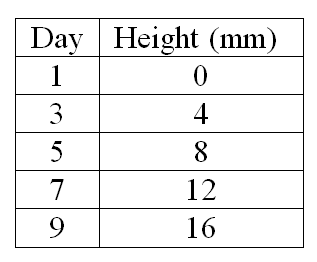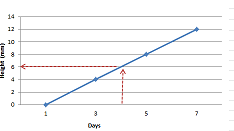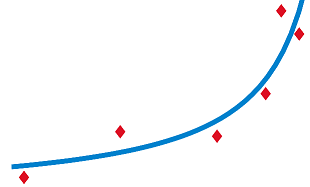# Linear Interpolation Formula

Interpolation Formula: The method of finding new values for any function using the set of values is done by interpolation. The unknown value on a point is found out using this formula. If linear interpolation formula is concerned then it should be used to find the new value from the two given points. If compared to Lagrange’s interpolation formula, the “n” set of numbers should be available and Lagrange’s method is to be used to find the new value.

Interpolation is the process of finding a value between two points on a line or curve. To help us remember what it means, we should think of the first part of the word, ‘inter,’ as meaning ‘enter,’ which reminds us to look ‘inside’ the data we originally had. This tool, interpolation, is not only useful in statistics, but is also useful in science, business or any time there is a need to predict values that fall within two existing data points.

## Linear Interpolation Formula

If the two known points are given by the coordinates {\displaystyle (x_{0},y_{0})}and {\displaystyle (x_{1},y_{1})}, the linear interpolant is the straight line between these points. For a value x in the interval {\displaystyle (x_{0},x_{1})}, the value y along the straight line is given from the equation of slopes

{\displaystyle {\frac {y-y_{0}}{x-x_{0}}}={\frac {y_{1}-y_{0}}{x_{1}-x_{0}}},}which can be derived geometrically from the figure on the right. It is a special case of polynomial interpolation with n = 1.

Solving this equation for y, which is the unknown value at x, gives

{\displaystyle y=y_{0}+(x-x_{0}){\frac {y_{1}-y_{0}}{x_{1}-x_{0}}}={\frac {y_{0}(x_{1}-x)+y_{1}(x-x_{0})}{x_{1}-x_{0}}},}which is the formula for linear interpolation in the interval {\displaystyle (x_{0},x_{1})}. Outside this interval, the formula is identical to linear extrapolation.

This formula can also be understood as a weighted average. The weights are inversely related to the distance from the end points to the unknown point; the closer point has more influence than the farther point. Thus, the weights are {\textstyle {\frac {x-x_{0}}{x_{1}-x_{0}}}}and {\textstyle {\frac {x_{1}-x}{x_{1}-x_{0}}}}, which are normalized distances between the unknown point and each of the end points. Because these sum to 1,

{\displaystyle y=y_{0}\left(1-{\frac {x-x_{0}}{x_{1}-x_{0}}}\right)+y_{1}\left(1-{\frac {x_{1}-x}{x_{1}-x_{0}}}\right)=y_{0}\left(1-{\frac {x-x_{0}}{x_{1}-x_{0}}}\right)+y_{1}\left({\frac {x-x_{0}}{x_{1}-x_{0}}}\right),}## Interpolation Formula Calculator

Solved Examples

Question 1: Using the interpolation formula, find the value of y at x = 8 given some set of values (2, 6), (5, 9) ?
Solution:

The known values are,x0=8,x1=2,x2=5,y1=6,y2=9y=y1+(xx1)(x2x1)×(y2y1)

y=6+((82)(52)×(96)

y = 6 + 6
y = 12

Linear interpolation is the simplest method of getting values at positions in between the data points. The points are simply joined by straight line segments.

Know the formula for the linear interpolation process. The formula is y = y1 + ((x – x1) / (x2 – x1)) * (y2 – y1), where x is the known value, y is the unknown value, x1 and y1 are the coordinates that are below the known x value, and x2 and y2 are the coordinates that are above the x value.

In the mathematical field of numerical analysis, interpolation is a method of constructing new data points within the range of a discrete set of known data points. … A few data points from the original function can be interpolated to produce a simpler function which is still fairly close to the original.

## Interpolation Formula Excel

Here’s an example that will illustrate the concept of interpolation. A gardener planted a tomato plant and she measured and kept track of its growth every other day. This gardener is a curious person, and she would like to estimate how tall her plant was on the fourth day.

Her table of observations looked like this:Based on the chart, it’s not too difficult to figure out that the plant was probably 6 mm tall on the fourth day. This is because this disciplined tomato plant grew in a linear pattern; there was a linear relationship between the number of days measured and the plant’s height growth. Linear pattern means the points created a straight line. We could even estimate by plotting the data on a graph.But what if the plant was not growing with a convenient linear pattern? What if its growth looked more like this?What would the gardener do in order to make an estimation based on the above curve? Well, that is where the interpolation formula would come in handy.

## Interpolation Formula Thermo

Linear interpolation has been used since antiquity for filling the gaps in tables. Suppose that one has a table listing the population of some country in 1970, 1980, 1990 and 2000, and that one wanted to estimate the population in 1994. Linear interpolation is an easy way to do this. The technique of using linear interpolation for tabulation was believed to be used by Babylonian astronomers and mathematicians in Seleucid Mesopotamia (last three centuries BC), and by the Greek astronomer and mathematician, Hipparchus (2nd century BC). A description of linear interpolation can be found in the Almagest (2nd century AD) by Ptolemy.

The basic operation of linear interpolation between two values is commonly used in computer graphics. In that field’s jargon it is sometimes called a lerp. The term can be used as a verb or noun for the operation. e.g. “Bresenham’s algorithm lerps incrementally between the two endpoints of the line.”

Lerp operations are built into the hardware of all modern computer graphics processors. They are often used as building blocks for more complex operations: for example, a bilinear interpolation can be accomplished in three lerps. Because this operation is cheap, it’s also a good way to implement accurate lookup tables with quick lookup for smooth functions without having too many table entries.

## Formula For Interpolation

Let us say that we have two known points x1,y1x1,y1 and x2,y2x2,y2.

Now we want to estimate what yy value we would get for some xx value that is between x1x1 and x2x2. Call this yy value estimate — an interpolated value.

Two simple methods for choosing yy come to mind. The first is see whether xx is closer to x1x1 or to x2x2. If xx is closer to x1x1 then we use y1y1 as the estimate, otherwise we use y2y2. This is called nearest neighbor interpolation.

The second is to draw a straight line between x1,y1x1,y1 and x2,y2x2,y2. We look to see the yy value on the line for our chosen xx. This is linear interpolation.

It is possible to show that the formula of the line between x1,y1x1,y1 and x2,y2x2,y2 is:

y=y1+(xx1)y2y1x2x1y=y1+(x−x1)y2−y1x2−x1

## Double Interpolation Formula

In order to perform a linear interpolation in Excel, we’ll use the equation below, where x is the independent variable and y is the value we want to look up:[Note: Want to learn even more about advanced Excel techniques? Watch my free training just for engineers. In the three-part video series I’ll show you how to easily solve engineering challenges in Excel. Click here to get started.]